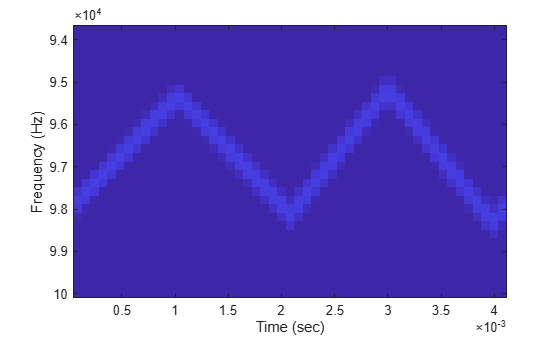## FMCW Waveforms

### Benefits of Using FMCW Waveform

Radar systems that use frequency-modulated, continuous-wave (FMCW) waveforms are typically smaller and less expensive to manufacture than pulsed radar systems. FMCW waveforms can estimate the target range effectively, whereas the simplest continuous-wave waveforms cannot.

FMCW waveforms are common in automotive radar systems and ground-penetrating radar systems.

### How to Create FMCW Waveforms

To create an FMCW waveform, use `phased.FMCWWaveform`. You can customize certain characteristics of the waveform, including:

• Sample rate.

• Period and bandwidth of the FM sweep. These quantities can cycle through multiple values during your simulation.

Tip

To find targets up to a given maximum range, `r`, you can typically use a sweep period of approximately `5*range2time(r)` or `6*range2time(r)`. To achieve a range resolution of `delta_r`, use a bandwidth of at least `range2bw(delta_r)`.

• Sweep shape. This shape can be sawtooth (up or down) or triangular.

Tip

For moving targets, you can use a triangular sweep to resolve ambiguity between range and Doppler.

`phased.FMCWWaveform` assumes that all frequency modulations are linear. For triangular sweeps, the slope of the down sweep is the opposite of the slope of the up sweep.

### Double Triangular Sweep

This example shows how to sample an FMCW waveform with a double triangular sweep in which the two sweeps have different slopes. Then, the example plots a spectrogram.

Note: This example runs only in R2016b or later. If you are using an earlier release, replace each call to the function with the equivalent `step` syntax. For example, replace `myObject(x)` with `step(myObject,x)`.

Create an FMCW waveform object for which the `SweepTime` and `SweepBandwidth` properties are vectors of length two. For each period, the waveform alternates between the pairs of corresponding sweep time and bandwidth values.

```st = [1e-3 1.1e-3]; bw = [1e5 9e4]; waveform = phased.FMCWWaveform('SweepTime',st,... 'SweepBandwidth',bw,'SweepDirection','Triangle',... 'SweepInterval','Symmetric','SampleRate',2e5,... 'NumSweeps',4);```

Compute samples from four sweeps (two periods). In a triangular sweep, each period consists of an up sweep and down sweep.

`x = waveform();`

Plot the spectrogram.

```[S,F,T] = spectrogram(x,32,16,32,waveform.SampleRate); image(T,fftshift(F),fftshift(mag2db(abs(S)))) xlabel('Time (sec)') ylabel('Frequency (Hz)')```